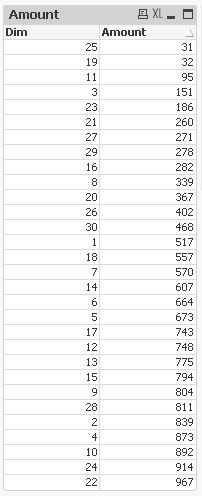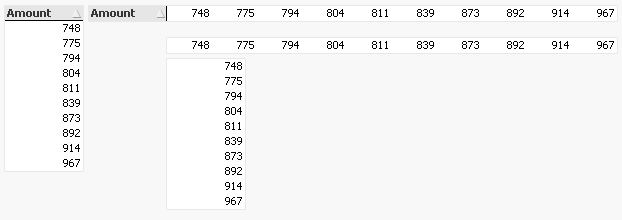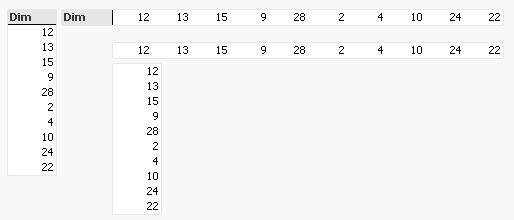QlikView Creating Analytics

Discussion Board for collaboration related to Creating Analytics for QlikView.

Highlighted
Contributor

List top n values in a text Object

Hi Gurus,

I have created a straight table which displays the Dimension values and sorted them as per the Rank calculated in the expression.

Now, I need to display all the top 10 values that I see in the Straight Table in one Text Object .

Using FirstSortedValue function in the Text Object, I could only display the top 1 or bottom 1 value.

-----

I also need to display Top 10, Bottom 10, and wrap all the rest of the Dimension values into 3 more Text Objects.

Tags (3)
5 RepliesMVP

Re: List top n values in a text Object

Maybe like this (assuming Sum(Value) is the expression used):

=Concat({<DimensionField = {"=Rank(Sum(Value))<=10"}>} DISTINCT DimensionField,', ', -Sum(Value) )

=Concat({<DimensionField = {"=Rank(-Sum(Value))<=10"}>} DISTINCT DimensionField,', ', -Sum(Value) )

=Concat({<DimensionField -= ( {"=Rank(Sum(Value))<=10"} +{"=Rank(-Sum(Value))<=10"} ) >}

DISTINCT DimensionField,', ', -Sum(Value) )

to list top 10, bottom 10 and all inbetween

Contributor

Re: List top n values in a text Object

Hi Swehl,

Thank you for the quick response.

I think i had it from your expression and realized that I may not have clearly stated my requirement.

I apologize.

Here's the error I am receiving in Text Object: Error in Expression. Nested aggregation not allowed

The Rank Expression that I am trying to use in the text object is a value = sum of 3 other ranks generated using 3 aggregated expression.

i.,e

RankA = Rank(sum(X))  * (.3)

+

RankB = Rank(AVG(Y)) * (.4)

+

RankC = Rank(sum(Z)) * (.3)

---

Please let me know if you rather prefer it to be created as a seperate discussionMVP

Re: List top n values in a text Object

Right, the sort weight needs advanced aggregation, like

=Concat(

{<DimensionField = {"=Rank(Sum(Value))<=10"}>}

DISTINCT

DimensionField,

', ',

Aggr(-Sum(Value),DimensionField)

)

What if you replace Rank(Sum(Value)) in above expression with your sum-of-three-ranks?

How do you get a total rank for your rank expression, BTW, are you using another rank function around the sum-of-three-ranks?MVP

Re: List top n values in a text Object

Hi,

if you already have the correct values in a straight table then maybe you can make it appear as a text box if that's your preferred visualization.

You could use dimension limits, hide caption, header rows and some columns and switch to horizontal presentation:using dimension limits:hiding caption and header row and using horizontal presentation.

hidden dimensionhidden expressionhope this helps

regards

Marco

Contributor

Re: List top n values in a text Object

Hi swehl,

Yes, i have to create a rank of the weighted sum of the three ranks ie., Rank(RankA*x% + RankB*y% + RankC*z%).

I made progress using the Advanced aggregation you suggested.

I was able to make some progress in displaying the top N values in one single 'text object'.

However, my requirement also needed me to display some additional dimension and expression values when I hover over each of the Dimension values in the 'text object'.

This leads me to create one 'text object' for each Rank. Then, i need to somehow use the 'Help Text' under 'Caption tab' of the 'text object' to display the required additional dimension and expression values relevant to the Dimension.

So, i gave up on this approach and started looking at creating one 'Straight Table' for each of the Dimension values that would look like a 'text object'  as Marco also suggested.

---

Hi Marco,

I started using this approach. Since I have to display more details when I hover over each of the Dimension values, I ended up creating about 300 straight tables with Conditional show/hide of each value ie., one for each Dimension value as I need to display all the Dimension values by Default. All these 300 straight tables are arranged in the required order of Top N , Everything in Between, Bottom N.

This has affected the Dashboard performance. The dashboard is not loading data unless i pass very little data.

So, even though I have visually what the user is looking to see, it just not effectively reloading data as needed.

--

I appreciate any advice and thank you for the help so far